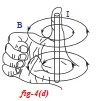# Perform an activity to show that current carrying wire produces magnetic field

### Magnetic field due to straight wire carrying current

Activity

Aim: To show that current carrying wire produces magnetic field.

Materials Required: Wooden plank, retort stand, 24 gauge copper wire, battery, switch, 6 to 10 compass, tableactivity current carrying wire generates magnetic field

Procedure:

1. Take a wooden plank and make a hole as shown in figure 4(a).
2. Place this plank on the table.
3. Now place a retort stand on the plank as shown in figure 4(a).
4. Pass 24 gauge copper wire through hole of the plank and rubber knob of the retort stand in such a way that the wire be arranged in a vertical position and not touch the stand.
5. Connect the two ends of the wire to a battery via switch.
6. Place 6 to 10 compass needles in a circular path around the hole so that its centre coincides with the hole.
7. Use 3 (or 9) volt battery in the circuit. Switch on.
8. Current flows through the wire.
9. Direction of magnetic field : If the current flow is vertically upwards (out of the page), the field lines are in anticlockwise direction, as shown in figure 4(b). If current flows into the page, in downward direction, the field lines are in clockwise direction as shown in figure 4(c).
10. Determination the direction of field lines:  It can be easily determined with right hand thumb rule. If we grab the current carrying wire with our right hand in such way that thumb is in the direction of current, then the curled fingers show the direction of the magnetic field as shown in figure 4(d).right hand thumb rule: direction of magnetic field in current carrying wire

Observation: We noticed that compass needles are directed as tangents to the circle.  It must be a circular line.

Conclusion: So we conclude that current carrying wire produces magnetic field  and magnetic field lines are closed lines. The magnetic field lines due to straight wire carrying the current is shown in figures 4(b) and 4(c). This can be verified by sprinkling iron filings around the wire when current flows in the wire.

Sources: Bajrai Online Solutions completed this lab activity from 10th Class textbook of SCERT.

—————–End Of Lab Activity————–

tags: describe an experiment to show that a magnetic field exerts a force on a current carrying conductor, force on a current carrying conductor in a magnetic field lab report, force on a current carrying conductor in a magnetic field notes, force on a conductor is unidirectional or bidirectional, explain an activity to show that a current carrying conductor experiences a force, the direction of the force on a current-carrying wire in an external magnetic field is, force on a current carrying conductor in a magnetic field class 10, when is the force experienced by a current carrying conductor placed in a magnetic field largest, FA III Physics project, FA III Physics lab activity, FA III School Project, Electromagnetism Lab Activity, Electromagnetism suggested experiments, School projects, complete school projects, Hyderabad directory, Business directory, bajraionline